Test: Basics of Digital Electronics- 2

# Test: Basics of Digital Electronics- 2

Test Description

## 20 Questions MCQ Test | Test: Basics of Digital Electronics- 2

Test: Basics of Digital Electronics- 2 for Electrical Engineering (EE) 2023 is part of Electrical Engineering (EE) preparation. The Test: Basics of Digital Electronics- 2 questions and answers have been prepared according to the Electrical Engineering (EE) exam syllabus.The Test: Basics of Digital Electronics- 2 MCQs are made for Electrical Engineering (EE) 2023 Exam. Find important definitions, questions, notes, meanings, examples, exercises, MCQs and online tests for Test: Basics of Digital Electronics- 2 below.
Solutions of Test: Basics of Digital Electronics- 2 questions in English are available as part of our course for Electrical Engineering (EE) & Test: Basics of Digital Electronics- 2 solutions in Hindi for Electrical Engineering (EE) course. Download more important topics, notes, lectures and mock test series for Electrical Engineering (EE) Exam by signing up for free. Attempt Test: Basics of Digital Electronics- 2 | 20 questions in 60 minutes | Mock test for Electrical Engineering (EE) preparation | Free important questions MCQ to study for Electrical Engineering (EE) Exam | Download free PDF with solutions
 1 Crore+ students have signed up on EduRev. Have you?
Test: Basics of Digital Electronics- 2 - Question 1

### Which of the following statements about digital systems are correct? 1. These are easier to design compared to analog systems. 2. These are highly affected by unwanted electrical signals. 3. Accuracy and precision of digital systems are greater when compared with analog systems. 4. The fabrication of digital ICs is complicated and costly than that of analog ICs. Choose the correct code from the given options:

Detailed Solution for Test: Basics of Digital Electronics- 2 - Question 1

The advantages of digital systems over analog systems are as follows:
1. Easier to design.
2. Less affected by noise (unwanted electrical signals).
3. More accuracy and precision.
4. Simple and economical fabrication into ICs.

Test: Basics of Digital Electronics- 2 - Question 2

### (1010.01)2 - (111.111)2 results into

Detailed Solution for Test: Basics of Digital Electronics- 2 - Question 2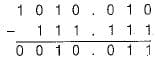In binary system, we take borrow as 2 for subtraction.
Thus, (1010.01)2 - (111.111)2 = (0010.011)2.

Test: Basics of Digital Electronics- 2 - Question 3

### Assertion (A): The advantage of performing subtraction by the complement method is reduction in the hardware. Reason (R): Instead of having separate digital circuits for addition and subtraction, only adding circuits are needed for subtraction by complement method.

Detailed Solution for Test: Basics of Digital Electronics- 2 - Question 3

Both assertion and reason are correct and reason is the correct explanation of assertion because when subtraction is performed using complement method, then instead of subtracting one number from other, the complement of the subtrahend is added to the minued, Thus, subtraction is also performed by adders only as in case of addition. Due to this reason the hardware requirement is reduced while performing subtraction by complement method,

Test: Basics of Digital Electronics- 2 - Question 4

The decimal equivalent of the hexadecimal number (A0F9.0EB)16 is

Detailed Solution for Test: Basics of Digital Electronics- 2 - Question 4

On multiplying the given hexadecimal number with the positional weightage, we get: (A0F9.0EB)16
= (10 x 163) + (0 x 162) + (15 x 161) + (9 x 160) + (0 x 16-1) + (14 x 16-2)+(11 x 16-3)
= 40960 + 0 + 240 + 9 + 0 + 0.0546 + 0.0026
= (41209.0572)10

Test: Basics of Digital Electronics- 2 - Question 5

If 100b = 1610, then the value of b would be equal to

Detailed Solution for Test: Basics of Digital Electronics- 2 - Question 5

On converting 100b to decimal, we have:
1 x b2 + 0 x b1 + 0 x b0 = 16
or, b2 = 16
or, b = 4

Test: Basics of Digital Electronics- 2 - Question 6

Consider the following series with a missing number;
10000,121,100, ?, 24, 22, 20
If in the above series, the same integer is expressed in different number systems, the missing number of the series would be equal to

Detailed Solution for Test: Basics of Digital Electronics- 2 - Question 6
• If we take the first number to be in binary (base 2), its equivalent will be (16)10 i.e. (10000)2 = (16)10.
• The next number is 121; it is equal to (16)10 in base 3 i.e. (121)3 = (16)10
• 100 is equal to (16)10 in base 4 i.e. (100)4 = (16)10.
• (31)s = (16)10
• (24)6 = (16)10
• (22)7 = (16)10
• (20)8 = (16)10

Hence, the missing number is 31 in base 5.

Test: Basics of Digital Electronics- 2 - Question 7

Each of the following arithmetic operations is correct in at least one number system:
(i) 1234 + 5432 = 6666 is valid in any number system with base ≥ 6.
(ii) √41 = 5 is valid in a number system with base 6.
(iii) 302/20 = 12.1 is valid in a number system with base 5.
Out of the above arithmetic operations:

Detailed Solution for Test: Basics of Digital Electronics- 2 - Question 7
• 1234 + 5432 = 6666
i.e.,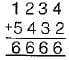Since the largest digit used is 6, this arithmetic operation is valid in any number system with base ≥ 7
• √41 = 5
Let the base be 5.
Then, its decimal equivalent operation is,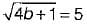or, 4b + 1 = 25 or, b = 6 Thus, the base is 6.
• 302/20 = 12.1
Expressing in decimal, we have:
(3b2 + 2)/2b = b + 2 + 1/b
or, 3b2 + 2 = 2b2 + 4b + 2
or, b2 - 4b = 0 or b = 4
(Neglecting b = 0)
Thus, the base is 4.
Hence, only (ii) is correct.
Test: Basics of Digital Electronics- 2 - Question 8

Consider the following statements:
1. In a self-complementing code, the code word for the 9's complement of N is the 1’s complement of the codeword for N.
2. Each one of the 4-bit BCD codes has 6 illegal states.
3. The Gray code cannot be used for mathematical operations.
4. Excess-3 code can be used for mathematical operations.
Which of the statements given above is/are correct?

Detailed Solution for Test: Basics of Digital Electronics- 2 - Question 8
• A code is said to be self-complementing, if the code word of the 9’s complement of N i.e. of (9-N) can be obtained from the code word of N by interchanging all the 0's and 1s. Thus, statement-1 is correct.
• There are six illegal combinations in 4-bit BCD codes which are 1010, 1011, 1100, 1101, 1110 and 1111 and hence they are not part of the 8421 BCD code system. Thus, statement-2 is also correct.
• The gray code can not be used for mathematical operations because it is non- weighted code. Thus, statement-3 is also correct.
• The excess-3 code is also a non-weighted code, but can be used for mathematical operations because it is a sequential code. Thus, statement-4 is also correct.
Test: Basics of Digital Electronics- 2 - Question 9

The ASCII code is a

Detailed Solution for Test: Basics of Digital Electronics- 2 - Question 9

The ASCII code is a alphanumeric code because it represents alphanumeric information i.e. fetters of the alphabet and decimal numbers as a sequence of 0s and 1s.

Test: Basics of Digital Electronics- 2 - Question 10

Assertion (A): The 2’s complement system has two zeros, a positive 0 (all 0s) and a negative 0 (all 1’s).
Reason (R): The complement of the complement gives the original number

Detailed Solution for Test: Basics of Digital Electronics- 2 - Question 10

The 1’s complement system has a disadvantage of having two zero representation i.e. a positive 0 (all 0s) and a negative 0 (all 1s). But, the 2's complement system has a unique zero.
Hence, assertion is not a correct statement.

Test: Basics of Digital Electronics- 2 - Question 11

Assertion (A): The computer method of division requires successive subtraction.
Reason (R): The computer method of multiplication requires repeated addition.

Test: Basics of Digital Electronics- 2 - Question 12

Assertion (A): Gray code is sometimes called reflective code.
Reason (R): The n least significant bits for 2n through (2n +1- 1) are the mirror images for 0 through (2n-1).

Test: Basics of Digital Electronics- 2 - Question 13

Which of the following 4-bit binary pairs does not forms a Gray code pair?

Detailed Solution for Test: Basics of Digital Electronics- 2 - Question 13

The Gray code is a cyclic code in which successive code words in this code differ in one bit position only i.e. it is a unit distance code. In options (a), (b) and (d) both the 4-bit binary numbers differ in one bit position only while in option (c) both the 4-bit binary numbers differs in two bit positions as shown below.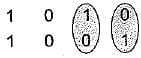Hence, option (c) does not forms a gray code pair of two given 4-bit binary numbers.

Test: Basics of Digital Electronics- 2 - Question 14

Match List-I (Data representation) with List-ll (Range with n-bits) and select the correct answer using the codes given below the lists:
List-1
A. Signed numbers in 1 ’s complement form
B. Signed magnitude
C. Unsigned magnitude
D. Signed numbers in 2’s complement form
List-ll
1. 0 to (2n - 1)
2. -(2n -1) to + (2n -1 - 1)
3. -(2n -1) to (2n -1)
4. -(2n -1 - 1) to + (2n -1 - 1)
Codes:
A B C D
(a) 3 3 1 2
(b) 1 4 3 4
(c) 2 4 1 3
(d) 4 4 1 2

Test: Basics of Digital Electronics- 2 - Question 15

A number is represented in 2’s complement as (1101)2. It’s equivalent decimal number is

Detailed Solution for Test: Basics of Digital Electronics- 2 - Question 15

2's complement representation of given number is (1101)2.
Since MSB is 1, therefore the required number must be negative.
1's complement of (1101)2 is (0010)2.
2's complement of 0010 - (0010)2 + (0001)2 = (0011)2.
Thus, the required number = -3 (Decimal equivalent of (0011)2 = 3).

Test: Basics of Digital Electronics- 2 - Question 16

Match List-I with List-ll and select the correct answer using the codes given below the lists:
List-I
A. (345)8
B. (11011.011)2
C. (47)16
List-II
1. (1B.6)16
2. (229)10
3. (107)8
4. (326)10
5. (D8.7)16
Code:
A B C
(a) 2 5 3
(b) 4 3 2
(c) 2 1 3
(d) 4 1 2

Detailed Solution for Test: Basics of Digital Electronics- 2 - Question 16
• (345)8 = (82 x 3) + (81 x 4) + (80 x 5) = (229)10 (Octal to decimal conversion
• (11011.011)2 =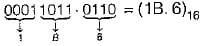(Binary to Hexadecimal conversion)
• (47)16 - (01000111)=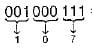= (107)8
(Hexadecimal to Binary and then to Octal Conversion)
Test: Basics of Digital Electronics- 2 - Question 17

Which of the following statements is/are correct in regard to excess-3 code?

Test: Basics of Digital Electronics- 2 - Question 18

Multiplication of two binary numbers A and B is 11011. If A = 011, then B is

Detailed Solution for Test: Basics of Digital Electronics- 2 - Question 18

Let,
B = (b3 b2b1b0)2
Now,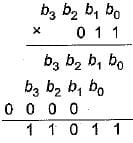Thus,
b0 = 1
b1 + b0 = 1   ∴ b1 = 0
b2 + b1 + 0 =  0   ∴ b2 = 0
b3 + 0 = 1   ∴ b= 1
Thus,
B = (b3b2b1b0)2
= (1001)2

Test: Basics of Digital Electronics- 2 - Question 19

Which of the following statement is not correct in regard to the “GRAY Code”?

Detailed Solution for Test: Basics of Digital Electronics- 2 - Question 19

The Gray code is not a BCD code.

Test: Basics of Digital Electronics- 2 - Question 20

Assertion (A): The “XS-3 code,” also called “Excess-3 code” is a non-weighted BCD code.
Reason (R): In XS-3 code, each binary code word is the corresponding 8421 code word plus 0011(3).

Information about Test: Basics of Digital Electronics- 2 Page
In this test you can find the Exam questions for Test: Basics of Digital Electronics- 2 solved & explained in the simplest way possible. Besides giving Questions and answers for Test: Basics of Digital Electronics- 2, EduRev gives you an ample number of Online tests for practice(Scan QR code)